GATE  >  Test: Open Channel Flow

# Test: Open Channel Flow

Test Description

## 20 Questions MCQ Test GATE Civil Engineering (CE) 2023 Mock Test Series | Test: Open Channel Flow

Test: Open Channel Flow for GATE 2023 is part of GATE Civil Engineering (CE) 2023 Mock Test Series preparation. The Test: Open Channel Flow questions and answers have been prepared according to the GATE exam syllabus.The Test: Open Channel Flow MCQs are made for GATE 2023 Exam. Find important definitions, questions, notes, meanings, examples, exercises, MCQs and online tests for Test: Open Channel Flow below.
Solutions of Test: Open Channel Flow questions in English are available as part of our GATE Civil Engineering (CE) 2023 Mock Test Series for GATE & Test: Open Channel Flow solutions in Hindi for GATE Civil Engineering (CE) 2023 Mock Test Series course. Download more important topics, notes, lectures and mock test series for GATE Exam by signing up for free. Attempt Test: Open Channel Flow | 20 questions in 60 minutes | Mock test for GATE preparation | Free important questions MCQ to study GATE Civil Engineering (CE) 2023 Mock Test Series for GATE Exam | Download free PDF with solutions
 1 Crore+ students have signed up on EduRev. Have you?
Test: Open Channel Flow - Question 1

### One kg force is equal to

Detailed Solution for Test: Open Channel Flow - Question 1

1 kg force= g N where g= acceleration due to gravity = 9.8 m/s2

Test: Open Channel Flow - Question 2

### A partially open sluice gate discharges water into a rectangular channel. The tail water depth in the channel is 3m and Froude number is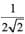. If a free hydraulic jump is to be formed at downstream of the sluice gate at vena contracta of the jet coming out from the sluice gate, the sluice gate opening should be (Coefficient of contraction Cc = 0.9)

Detailed Solution for Test: Open Channel Flow - Question 2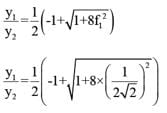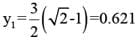Opening the sluice gate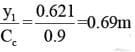Test: Open Channel Flow - Question 3

### On sag (or valley) curves the available sight distance is determined based on :

Detailed Solution for Test: Open Channel Flow - Question 3

In valley curves two conditions are required-
Safety Criteria  In which the driver should have adequate light during night.
Comfort Criteria In which the change in centrifugal acceleration is limited upto a certain level.

Test: Open Channel Flow - Question 4

The sequent depths in a hydraulic jump formed in a 5m wide rectangular channel are 0.2m and 1m. The discharge in the channel is:

Detailed Solution for Test: Open Channel Flow - Question 4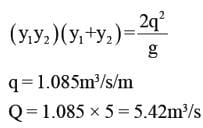Test: Open Channel Flow - Question 5

Maximum size of a fillet weld for a plate of square edge is

Detailed Solution for Test: Open Channel Flow - Question 5

The maximum size of a fillet weld is obtained by subtracting 1.5 mm from the thickness of the thinner member to be jointed. This specification limits the size of the fillet weld so that total strength may be developed without overstressing the adjacent metal.

Test: Open Channel Flow - Question 6

The number of simultaneous equations to be solved in the slope deflection method, is equal to :

Detailed Solution for Test: Open Channel Flow - Question 6

For every joint a slope deflection equation will be written of the form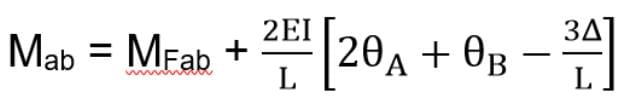Mab = MFab + 2EI/L [2θ_A+θ_B-3Δ/L]
Thus, number of simultaneous equations available will be equal to the number of joints.

Test: Open Channel Flow - Question 7

A rectangular channel carries a certain flow for which the alternate depths are found to be 3m and 1m. The critical depth (in m) for this flow is:

Detailed Solution for Test: Open Channel Flow - Question 7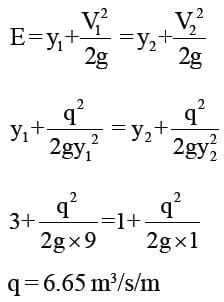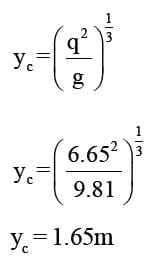Test: Open Channel Flow - Question 8

In a triangular channel, the critical depth is 2m. The corresponding least possible specific energy is:

Detailed Solution for Test: Open Channel Flow - Question 8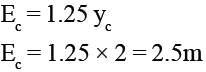Test: Open Channel Flow - Question 9

If the Froude number of flow in a rectangular channel at a depth of flow of y0 is F0, then what is yc/y0 equal to?

Detailed Solution for Test: Open Channel Flow - Question 9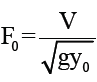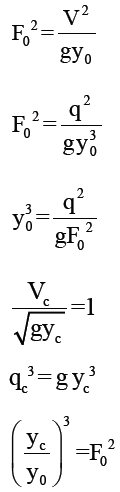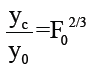Test: Open Channel Flow - Question 10

A rectangular channel 3m wide carries uniform flow of water at a rate of 7 cumec/sec at a depth of 1.5m. If there is a local rise of 0.5m in the bed, calculate the change in upstream depth of flow?

Detailed Solution for Test: Open Channel Flow - Question 10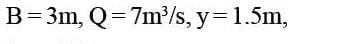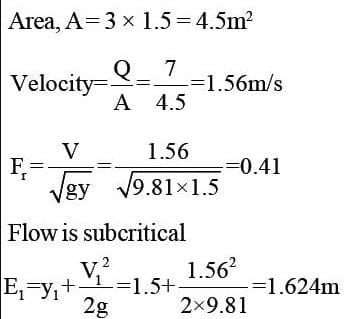As bed level  rises by 0.5m, specific energy at section  2 decreasees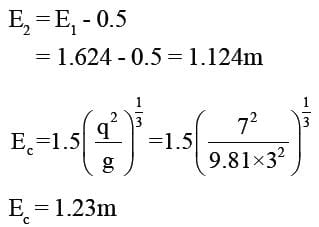Since, E2<Ec hence there is change in upstream depth  condition.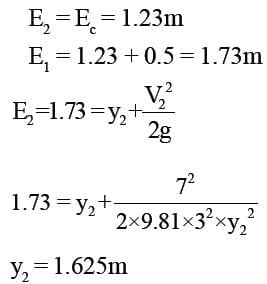Increase in upstream depth
= 1.625 - 1.5
= 0.125m

Test: Open Channel Flow - Question 11

A wide rectangular channel carries discharge of 6 m3/s per meter width. The channel has a bed slope of 0.009 and Manning's roughness coefficient, n = 0.015. At a certain section of the channel, the flow depth is 1.5m. What gradually varied flow profile exist at this section ?

Detailed Solution for Test: Open Channel Flow - Question 11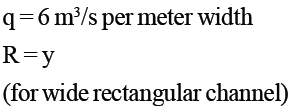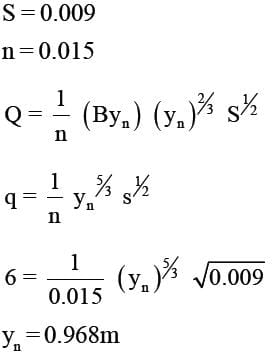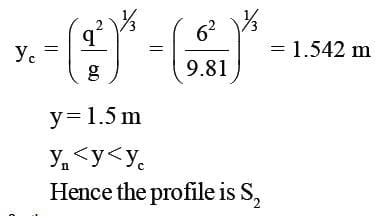Test: Open Channel Flow - Question 12

In a hydraulic jump occurring in a rectangular channel of 3m width, the discharge is 8 m3/s, and the depth of flow before jump is 0.25m. Find the energy loss in the jump ?

Detailed Solution for Test: Open Channel Flow - Question 12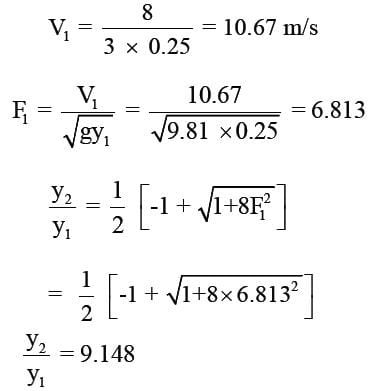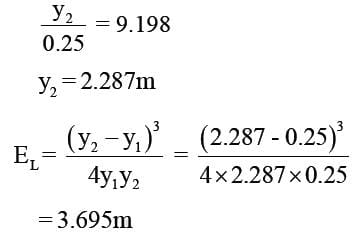Test: Open Channel Flow - Question 13

The sequent depth ratio in a hydraulic jump formed in a horizontal rectangular channel is 16.48. The Froude number of the super critical stream is :

Detailed Solution for Test: Open Channel Flow - Question 13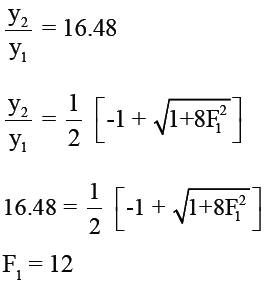Test: Open Channel Flow - Question 14

A mild slope channel enters a lake with a sudden drop in bed. If the depth of water in the lake measured above the channel bed at its outlet yL is greater than the critical depth, then the depth of flow in the canal at the outlet yd :

Test: Open Channel Flow - Question 15

Water emerges from an ogee spillway with velocity 13.72 m/s and depth = 0.3 m at its toe. The tail water depth required to form a hydraulic jump at the toe is

Detailed Solution for Test: Open Channel Flow - Question 15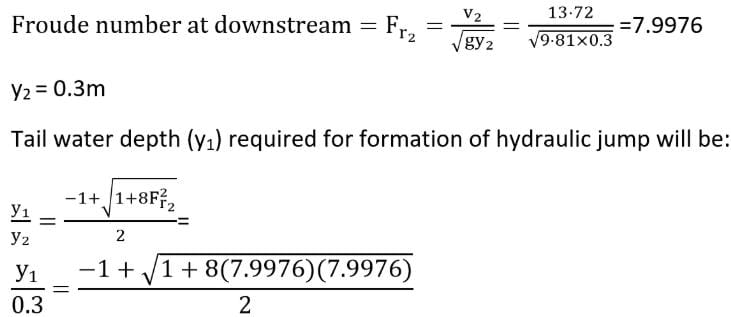y_1 = 3.2464 m

Test: Open Channel Flow - Question 16

The ratio of unconfined compressive strength of an undisturbed sample of soil to that of a remoulded sample, at the same water content, is known as :

Detailed Solution for Test: Open Channel Flow - Question 16

Sensitivity is the ratio of unconfined compressive strength of cement of an undisturbed sample to unconfined compressive strength of remoulded sample.
Activity is the ratio of plasticity index to percentage of particles finer than 2 micron.

Test: Open Channel Flow - Question 17

In a channel the bed slope changes from mild slope to steep slope. The resulting GVF profiles are :

Detailed Solution for Test: Open Channel Flow - Question 17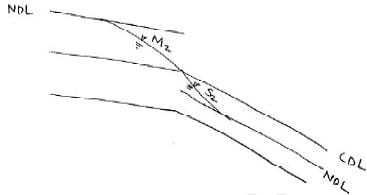Flow profile is (M2,S2)

Test: Open Channel Flow - Question 18

In a wide river the depth of flow at a section is 3m, S0 = 1 in 5000 and q = 3m3/s per meter width. If the Chezy formula with C = 70 is used, the water surface slope relative to the bed at the section is:

Detailed Solution for Test: Open Channel Flow - Question 18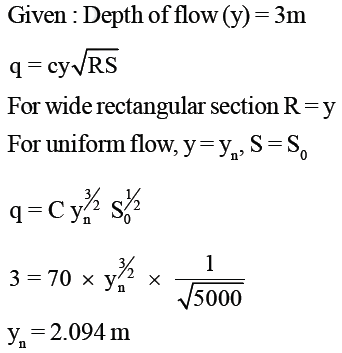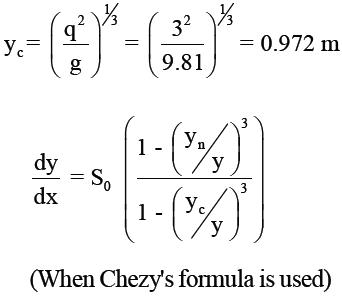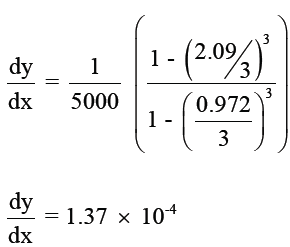Test: Open Channel Flow - Question 19

At critical depth :

Detailed Solution for Test: Open Channel Flow - Question 19

At critical depth discharge is maximum for a given specific energy. Also, it can be said as that specific energy is minimum for a given discharge. Also, at critical depth Froude number is equal to 1.

Test: Open Channel Flow - Question 20

A triangular channel of side slope 3:1 carries water at a flow rate of 10m3 /s. If the depth of the flow is 1.32m and the channel is laid on slope of 0.0018, find Manning’s n.

Detailed Solution for Test: Open Channel Flow - Question 20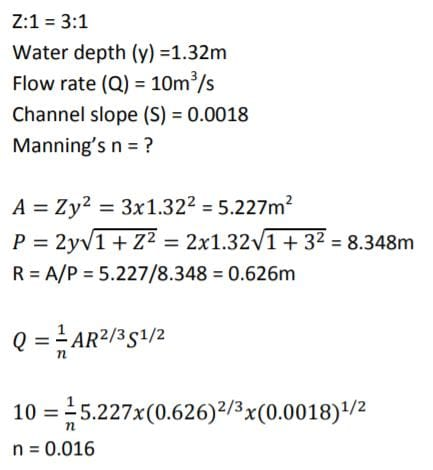## GATE Civil Engineering (CE) 2023 Mock Test Series

26 docs|292 tests
 Use Code STAYHOME200 and get INR 200 additional OFF Use Coupon Code
Information about Test: Open Channel Flow Page
In this test you can find the Exam questions for Test: Open Channel Flow solved & explained in the simplest way possible. Besides giving Questions and answers for Test: Open Channel Flow, EduRev gives you an ample number of Online tests for practice

## GATE Civil Engineering (CE) 2023 Mock Test Series

26 docs|292 tests